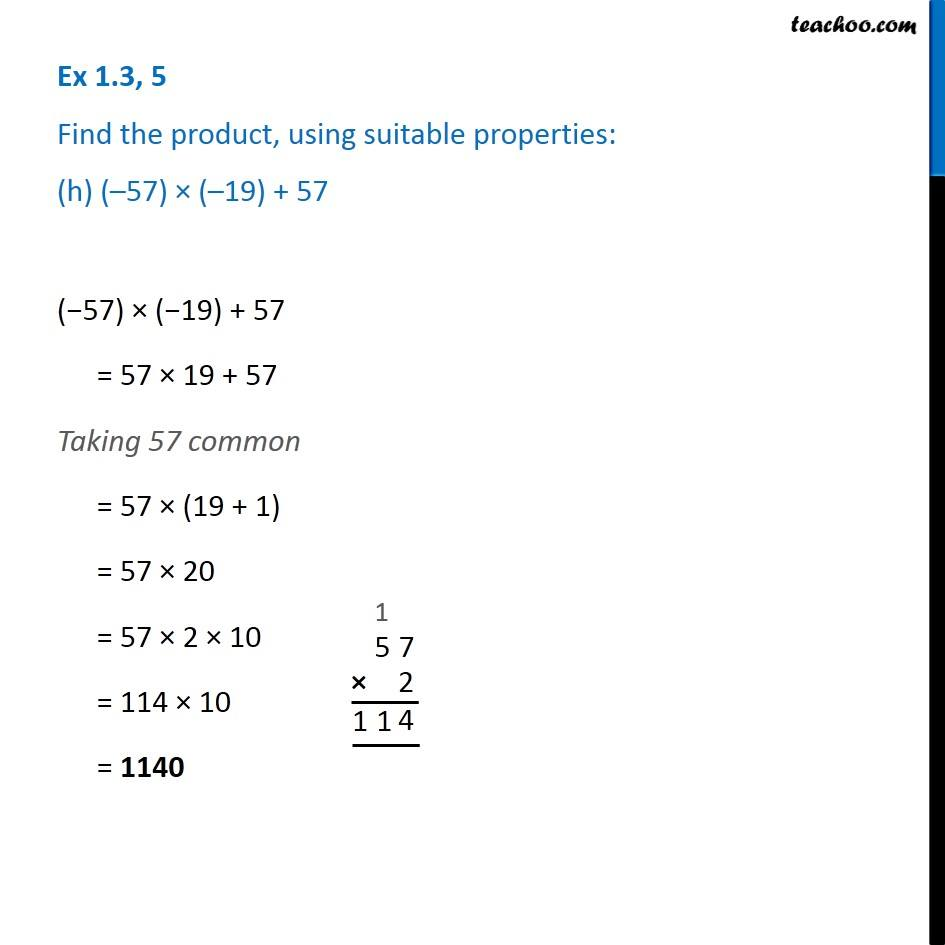Ex 1.2

Chapter 1 Class 7 Integers
Serial order wiseLearn in your speed, with individual attention - Teachoo Maths 1-on-1 Class

### Transcript

Ex 1.2, 5 Find the product, using suitable properties: (h) (–57) × (–19) + 57 (−57) × (−19) + 57 = 57 × 19 + 57 Taking 57 common = 57 × (19 + 1) = 57 × 20 = 57 × 2 × 10 = 114 × 10 = 1140